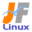Linux Kernel 2.6 Documentation: /usr/src/linux/Documentation/filesystems/sysfs.txt

# filesystems/sysfs.txt

sysfs \$B%U%!%\$%k%7%9%F%`\$N@bL@(B [\$B%W%l%\$%s%F%-%9%HHG(B]
• \$B86Cx:n
• \$BK]Lu
• \$B%P!<%8%g%s(B: 2.6.13
• \$BK]LuF|;~(B: 2005/08/28
```

==================================
\$B\$3\$l\$O!"(B
linux-2.6.13-rc5/Documentation/filesystems/sysfs.txt \$B\$NOBLu(B
\$B\$G\$9!#(B
\$BK]LuCDBN!'(B JF \$B%W%m%8%'%/%H(B < http://www.linux.or.jp/JF/ >
\$B99?7F|(B \$B!'(B 2005/8/28
\$B86Cx:n\:Y>pJs\$O!"(BDocumentation/kobject.txt \$B\$rFI\$s\$G\$/\$@\$5\$\$!#(B

Using sysfs

sysfs \$B\$r;H\$&(B
~~~~~~~~~~~

sysfs is always compiled in. You can access it by doing:

sysfs \$B\$O>o\$K(B (\$B%+!<%M%k\$K(B) \$BAH\$_9~\$^\$l\$^\$9!#@\E*\$J;2>H\$,\$G\$-\$k\$h\$&\$K\$7\$^\$9!#(B

Attributes

\$BB0@-(B
~~~~~~~~~~

Attributes can be exported for kobjects in the form of regular files in
the filesystem. Sysfs forwards file I/O operations to methods defined
for the attributes, providing a means to read and write kernel
attributes.

\$BB0@-\$O!"%U%!%\$%k%7%9%F%`Fb\$N\$-\$^\$C\$?%U%!%\$%k\$N7A\$r\$7\$F\$\$\$k(B kobject \$B\$N\$?\$a\$K(B
\$B=hM}\$rE>Aw\$9\$k\$3\$H\$G!"%+!<%M%kB0@-\$NFI\$_=q\$-Aw\$9\$k\$?\$a\$N!"(Bsysfs \$B=hM}0l<0\$rl=j(B) \$B\$r4{\$KDj5A\$7\$F\$\$\$k\$O\$:\$G\$9(B ]

When a file is read or written, sysfs calls the appropriate method
for the type. The method then translates the generic struct kobject
and struct attribute pointers to the appropriate pointer types, and
calls the associated methods.

\$B%U%!%\$%k\$,FI\$_=q\$-\$5\$l\$k\$H\$-!"(Bsysfs \$B\$O!"\$=\$N%?%\$%W\$KE,@Z\$J%a%=%C%I\$r8F\$S=P\$7\$^\$9!#(B
\$B%a%=%C%I\$O!"HFMQ(B kobject \$B9=B\$BN\$*\$h\$S(B \$BB0@-%]%\$%s%?9=B\$BN\$rE,@Z\$J%]%\$%s%?%?%\$%W\$K(B
\$BJQ49\$7\$?\$"\$H\$G!"4XO"\$9\$k%a%=%C%I\$r8F\$S\$^\$9!#(B

To illustrate:

\$B%3!<%I\$r=q\$\$\$F\$_\$^\$7\$g\$&(B:

#define to_dev_attr(_attr) container_of(_attr,struct device_attribute,attr)
#define to_dev(d) container_of(d, struct device, kobj)

static ssize_t
dev_attr_show(struct kobject * kobj, struct attribute * attr, char * buf)
{
struct device_attribute * dev_attr = to_dev_attr(attr);
struct device * dev = to_dev(kobj);
ssize_t ret = 0;

if (dev_attr->show)
ret = dev_attr->show(dev,buf);
return ret;
}

\$BB0@-%G!<%?\$rFI\$_=q\$-\$9\$k(B
~~~~~~~~~~~~~~~~~~~~~~~~~~~~~~

To read or write attributes, show() or store() methods must be
specified when declaring the attribute. The method types should be as
simple as those defined for device attributes:

\$BB0@-\$rFI\$_=q\$-\$9\$k\$K\$O!"B0@-\$r@k8@\$9\$k\$H\$-\$K!"(Bshow() \$B\$^\$?\$O!"(Bstore() \$B%a%=%C%I\$,!"(B
\$B%3!<%I\$K=q\$+\$l\$F\$\$\$J\$1\$l\$P\$J\$j\$^\$;\$s!#(B

ssize_t (*show)(struct device * dev, char * buf);
ssize_t (*store)(struct device * dev, const char * buf);

IOW, they should take only an object and a buffer as parameters.

\$B8@\$\$\$+\$(\$l\$P(B (IOW = in other words)\$B!"\$=\$l\$i\$O%Q%i%a!<%?\$H\$7\$F\$R\$H\$D\$N%*%V%8%'%/%H(B
\$B\$*\$h\$S!"\$R\$H\$D\$N%P%C%U%!\$@\$1\$r\$l\$N\$?\$a\$K!"%a%=%C%I\$r3N\$`\$H\$-\$K!"%U%!%\$%kA4BN\$NG\$0U\$N0LCV\$+\$iItJ,E*\$KFI\$_=P\$7\$?\$j!"(B
\$B0\F0\$9\$k\$3\$H\$r2DG=\$K\$7\$^\$9!#(B

- On write(2), sysfs expects the entire buffer to be passed during the
first write. Sysfs then passes the entire buffer to the store()
method.

- write(2) \$B\$K\$*\$\$\$F!"(Bsysfs \$B\$O:G=i\$N=q\$-9~\$_Cf\$K!"%P%C%U%!A4BN\$,EO\$5\$l\$k\$3\$H\$rI,MW\$H(B
\$B\$7\$^\$9!#\$=\$N\$"\$H\$G!"(Bsysfs \$B\$O!"(Bstore() \$B%a%=%C%I\$K%P%C%U%!A4BN\$rEO\$7\$^\$9!#(B

When writing sysfs files, userspace processes should first read the
entire file, modify the values it wishes to change, then write the
entire buffer back.

sysfs \$B%U%!%\$%k\$K=q\$-9~\$_Cf!"%f!<%66u4V\$N=hM}\$O!"\$O\$8\$a\$K%U%!%\$%kA4BN\$rFI\$_9~\$_!"(B
\$BJQ99\$7\$?\$\$CM\$rJT=8\$7!"\$=\$N\$"\$H\$G!"%P%C%U%!A4BN\$r8e\$m\$K=q\$-=P\$5\$J\$1\$l\$P\$J\$j\$^\$;\$s!#(B

Attribute method implementations should operate on an identical
buffer when reading and writing values.

\$BB0@-%a%=%C%I\$O!"CM\$NFI\$_=q\$-\$r\$R\$H\$D\$N%P%C%U%!>e\$G=hM}\$9\$ko\$K!"(BPAGE_SIZE \$B%P%\$%H\$G\$9!#(Bi386 \$B\$G\$O!"(B4096 (\$B%P%\$%H(B) \$B\$G\$9!#(B

- show() methods should return the number of bytes printed into the
buffer. This is the return value of snprintf().

- show() \$B%a%=%C%I\$O!"%P%C%U%!\$K=q\$-9~\$s\$@%P%\$%H?t\$rJV\$5\$J\$1\$l\$P\$J\$j\$^\$;\$s!#(B
\$B\$3\$l\$O!"(Bsnprintf() \$B\$NLa\$jCM\$G\$9!#(B

- show() should always use snprintf().

- show() \$B\$O>o\$K!"(Bsnprintf() \$B\$r;H\$o\$J\$1\$l\$P\$J\$j\$^\$;\$s!#(B

- store() should return the number of bytes used from the buffer. This
can be done using strlen().

- store() \$B\$O!"%P%C%U%!\$+\$i;H\$C\$?%P%\$%H?t\$rJV\$5\$J\$1\$l\$P\$J\$j\$^\$;\$s!#(B
\$B\$3\$l\$O!"(Bstrlen() \$B\$r;H\$C\$F\$G\$-\$^\$9!#(B

- show() or store() can always return errors. If a bad value comes
through, be sure to return an error.

- show() \$B\$d!"(Bstore() \$B\$O!">o\$K%(%i!<\$rJV\$;\$^\$9!#IT@5\$JCM\$,\$-\$?\$H\$-\$K\$O!"I,\$:%(%i!<\$r(B
\$BJV\$9\$3\$H!#(B

- The object passed to the methods will be pinned in memory via sysfs
referencing counting its embedded object. However, the physical
entity (e.g. device) the object represents may not be present. Be
sure to have a way to check this, if necessary.

- \$B%a%=%C%I\$KEO\$5\$l\$?%*%V%8%'%/%H\$O!"(Bsysfs \$B\$N4IM}\$9\$kKd\$a9~\$_%*%V%8%'%/%H\$N;2>H%+%&%s%H(B
\$B?t\$K\$h\$C\$F!"%a%b%jFb\$K%T%s;_\$a(B (\$B8GDj(B) \$B\$5\$l\$^\$9!#(B
\$B\$7\$+\$7!"%*%V%8%'%/%H\$,I=\$9!"J*M}E*o\$K4JC1\$J(B (\$B\$=\$7\$FC1=c\$J(B) \$BA0\$r%;%C%H\$G\$-\$J\$\$\$3\$H\$KCm0U\$7\$F\$/\$@\$5\$\$!#(B)

Top Level Directory Layout

\$B:G>e0L%G%#%l%/%H%j%l%\$%"%&%H(B
~~~~~~~~~~~~~~~~~~~~~~~~~~

The sysfs directory arrangement exposes the relationship of kernel
data structures.

sysfs \$B%G%#%l%/%H%j\$NG[CV\$O!"%+!<%M%k%G!<%?9=B\$\$NO"7H\$rI=\$7\$^\$9!#(B

The top level sysfs diretory looks like:

\$B:G>e0L\$N(B sysfs \$B%G%#%l%/%H%j\$O!"\$3\$N\$h\$&\$K8+\$(\$^\$9(B:

block/
bus/
class/
devices/
firmware/
net/

devices/ contains a filesystem representation of the device tree. It maps
directly to the internal kernel device tree, which is a hierarchy of
struct device.

devices/ \$B\$K\$O!"%G%P%\$%9%D%j!<\$r!"%U%!%\$%k%7%9%F%`I=8=\$K\$7\$?\$b\$N\$,\$"\$j\$^\$9!#(B
device \$B9=B\$BN\$N3,AX\$G\$"\$k!"FbItE*\$J%+!<%M%k%G%P%\$%9%D%j!<\$KD>@\%^%C%W\$5\$l\$F\$\$\$^\$9!#(B

bus/ contains flat directory layout of the various bus types in the
kernel. Each bus's directory contains two subdirectories:

bus/ \$B%+!<%M%kFb\$K\$*\$1\$k\$5\$^\$6\$^\$J%P%9%?%\$%W\$NJ?C3\$J%G%#%l%/%H%j%l%\$%"%&%H\$r3JG<\$7\$F\$\$\$^\$9!#(B
\$B\$=\$l\$>\$l\$N%P%9\$N%G%#%l%/%H%j\$O!"\$U\$?\$D\$N%5%V%G%#%l%/%H%j\$r3JG<\$7\$F\$\$\$^\$9(B:

devices/
drivers/

devices/ contains symlinks for each device discovered in the system
that point to the device's directory under root/.

devices/ \$B\$O!"(Broot/ \$B2<\$K\$"\$k%G%P%\$%9\$N%G%#%l%/%H%j\$r;X\$9!"%7%9%F%`Fb\$GH/8+\$5\$l\$?(B
\$B\$=\$l\$>\$l\$N%G%P%\$%9\$X\$N%7%s%\%j%C%/%j%s%/\$r3JG<\$7\$F\$\$\$^\$9!#(B

drivers/ contains a directory for each device driver that is loaded
for devices on that particular bus (this assumes that drivers do not
span multiple bus types).

drivers/ \$B\$O!"FCDj\$N%P%9(B (\$B\$3\$l\$O!"%I%i%\$%P\$,J#?t\$N%P%9%?%\$%W\$K\$^\$?\$,\$i\$J\$\$\$H2>Dj\$7\$F\$\$\$^\$9(B)
\$B>e\$N%G%P%\$%9MQ\$K%m!<%I\$5\$l\$?!"\$=\$l\$>\$l\$N%G%P%\$%9%I%i%\$%P\$N\$?\$a\$N%G%#%l%/%H%j\$r3JG<\$7\$F\$\$\$^\$9!#(B

Documentation/driver-model/.

\$B%I%i%\$%P%b%G%kFCM-\$NFCD'\$K4X\$9\$k>\:Y\$O!"(BDocumentation/driver-model/ \$B\$K\$"\$j\$^\$9!#(B

TODO: Finish this section.

TODO: \$B\$3\$N%;%/%7%g%s\$r40@.\$9\$k(B

Current Interfaces

\$B8=:_\$N%\$%s%?%U%'!<%9(B
~~~~~~~~~~~~~~~~~~

The following interface layers currently exist in sysfs:

\$B8=:_!"(Bsysfs \$B\$K\$O!"

Linux \$B%+!<%M%k(B 2.6 \$BIUB0J8=q0lMw\$XLa\$k(B

JF Project \$B%a%\$%s%Z!<%8\$X(B
JF Project \$BA4HL\$K4X\$o\$k\$40U8+\$4MWK>\$O(B jf@listserv.linux.or.jp \$B\$^\$G\$*4j\$\$\$7\$^\$9(B.

```